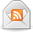# How to Make Sense of Interest Rates, APYs and APRs

Posted by Amanda on April 29, 2015

Have you noticed that interest is not interest is not interest? There are so many different types of interest rates for finances that it gets a bit confusing (even for me). Honestly, before writing this article I didn’t know what each type of interest rate meant. For example, what is the difference between an APR and APY? Would you rather pay a compound interest rate, or simple interest rate on your debt?

I am going to break down the different interest rates in two different categories: interest rates paid to you (savings), and interest rates paid by you (debt).

### Common Savings Interest Rates

This is the fun category, because it’s money being paid to you for keeping your savings in an account. Here are some common savings interest rates:

• Compound Interest: Compound interest means that each time interest is calculated, it is added onto the principal amount. Then when interest is calculated next, it is calculated on the principal + the interest that was added in last time. The more frequently that interest is set to compound, the more interest you will earn on your money.
• Annual Percentage Yield (APY): APY is the amount of interest you earn over a year. However, this interest rate takes into account compounding. This is because interest is typically earned monthly (sometimes quarterly) on savings account. Banks take the interest rate, divide it by 12 (or 4 if quarterly), and multiply your savings by that number in order to calculate your savings for a month. Then that amount is added onto your principal, so that the next month you earn money on your principal + interest.

### Common Debt Interest Rates

These are the not-so-fun interest rates because they calculate how much money we owe our lenders on top of the actual debt we have. Read: servicing your debt instead of paying it down aggressively is quite expensive. Here are some common debt interest rates:

• Introductory Interest Rate (aka Promotional Interest Rate): Many credit cards offer an introductory or promotional interest rate. These are teaser rates that are generally very good so that you will sign up for the offer. Beware that at the end of a teaser rate, the real interest rate will rise (and sometimes quite substantially).
• Fixed Interest Rate: Fixed interest rates are ones that will remain the same for the duration of the agreement. This is generally a good thing as you can count on how much your interest will be each month. Many mortgages offer fixed mortgage rates.
• Variable Interest Rate: These interest rates vary according to whatever factor it is tied to. The factor could be an underlying benchmark interest rate or index that changes periodically. If interest rates are generally low, then it’s a good time for people who have variable interest rates. Unfortunately, when interest rates spike, then those with variable interest rates will have more of their monthly payments go towards interest instead of principal.
• Simple Interest: Simple interest only calculates on the principal amount. Interest does not accrue on interest debt accumulated.
• Annual Percentage Rate (APR):This tells you what your interest is for an entire year (annualized) versus what you would pay in interest each month. Of course, to figure out your monthly rate, just divide this by 12.
• Nominal APR:This is a simple interest rate in its calculation over one year. This typically does not show the real cost, as it does not include any fees that are assessed.
• Effective APR: This is an interest rate calculation that includes any fees (such as participation fees, loan origination fees, monthly service charges, or late fees) plus compound interest. The effective APR is calculated using one of three ways: compounding the interest rate for each year, without considering fees; origination fees are added to the balance due, and the total amount is treated as the basis for computing compound interest; and the origination fees are amortized (due in the first payment), and then a second loan is the rest (a long-term loan).
• Amortized Interest: This is what you encounter with mortgages and auto loans. Each month, the payments you send are calculated so that you pay all of the interest due for that month plus a small amount towards principal. This means that every month you pay slightly more towards principal than towards interest until eventually the loan is paid off.

Hopefully this knowledge will equip you better to make decisions on debt repayment and loans. If nothing else, at least your eyes won’t glaze over the next time someone starts to talk about interest rates with you.

### More Financial Calculations